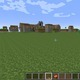# Unresolved Detail In Plotted Equations

Sometimes the calculator detects that an equation is too complicated to plot perfectly in a reasonable amount of time. When this happens, the equation is plotted at lower resolution.

256 out of 283 found this helpful
Have more questions? Submit a request

•Ezra Seidel

What have you done?!

•SAurav TYagi

$y=\mod \left(x^2,y^2\right)$

•Pedro Gómez

i just got crazy

•Vanderkeystone

Here's a simple one: x^(log_x y)=y
aka
x^{\log _xy}=y

•Artur Welp

x=sec((x)²+(y)²)

•JDLENL

\sin xy=\frac{\tan y+x^2}{x!!y^{\frac{2\pi }{3}}+\left|\sqrt{xy}\right|}

•Euan Zhang

y = |y| tan x

•Euan Zhang

y = |y| tan x

•11003105

um try this:

floor(2x)+floor(2y)>=floor(x)+floor(y)+floor(x+y)

•JDLENL

\sqrt{\frac{\csc x}{\sin x^2}}^{-1}

•Ethan LI

\frac{x^{\frac{2\cdot \sqrt{x}}{6\sqrt{y}}}}{x^{3\sqrt{\frac{xyy}{\frac{yxx}{xyy}}}}}=y^{\frac{\sqrt{\left(\sqrt{x\sqrt{y+\sqrt{x^{2^{2\cdot \sqrt{x}}}x}}}\right)}}{\left(\sqrt{x^{\sqrt{\frac{2\cdot \sqrt{x}}{6\sqrt{y}}}}}\cdot \frac{0.3xxx}{0.3y}\right)}}\cdot \frac{\left(4x^{-2}\sqrt{x^{-y}-\frac{x}{y^{222}}}\right)}{xy^{3\sqrt{xx}}}
does not show the saying but works the same.

•JDLENL

\sqrt{x\sqrt{x\sqrt{x\sqrt{x\sqrt{x\sqrt{x\sqrt{x\sqrt{x\sqrt{x\sqrt{x\sqrt{x\sqrt{x\sqrt{x\sqrt{x\sin \left(x\right)}}}}}}}}}}}}}}

•Phillip Varnston

I have a question: I was simplifying an equation someone commented on here last June and on one side of the equation I had (1/ln(x/y))/(1/ln(x/y)). Now, naturally, this would simplify to 1 with no change to the graph, but when I do that, it flips on the y-axis. Is this correct, or is it just an error in the calculator?

(Now, before you call me stupid, I'm a junior in High School who is currently taking Algebra 2 and hasn't taken a day in any form of calculus. I'm just fiddling around with the graphing calculator.)

Here's the unsimplified equation:
\frac{\frac{\tan \left(\cos \left(\sin x\right)\right)}{\left(\cos xy\right)\cdot \frac{\ln \frac{y}{x}}{\cos xy}}}{\frac{\tan \left(\cos \left(\sin x\right)\right)}{\left(\cos xy\right)\cdot \frac{\ln \frac{y}{x}}{\cos xy}}\cdot \frac{\frac{\tan \left(\cos \left(\sin y\right)\right)}{\left(\cos xy\right)\cdot \frac{\sin xy}{x}}}{\left(\cos xy\right)\cdot \frac{\sin xy}{x}}}=\frac{\frac{\tan \left(\cos \left(\sin y\right)\right)}{\left(\cos xy\right)\cdot \frac{\sin xy}{x}}}{\left(\cos xy\right)\cdot \frac{\sin xy}{x}}

And this is the most I can simplify from it:
\frac{\frac{1}{\ln \frac{y}{x}}}{\frac{1}{\ln \frac{y}{x}}}=\left(\frac{\tan \left(\cos \left(\sin y\right)\right)}{\left(\left(\cos xy\right)\cdot \frac{\sin xy}{x}\right)^2}\right)^2

•Ezra Seidel

y=\sum _{n=x}^yn

•Dylan Honors

@Phillip Varnston the natural log of a negative number is undefined. this creates a domain restriction in the graph. removing the logs allows negative values of x to be defined.

•. 狼丸

https://www.desmos.com/calculator/p2yid2mrzz
I think the plotter hates me now.

•Butter Gamer (Blake)

y^x=3^(2x+1)

•Arnav Thakar

\tan y^a=\tan x^a

Where you click the play button for a

•Brandon Shane

x^n+y^m\ =\ x^{\left(n\ +\ \frac{\log \left(1\ +\ x^{\left(\frac{m\log \left(y\right)}{\log \left(x\right)}-n\right)}\right)}{\log \left(x\right)}\right)}

•Splendormite

y=\sin x\cdot \frac{x}{y}

•Will Moreton

5=(1-acsc((x*4)^2+(y*4)^2))/1000

•Christian Grody

Well this graphs sinx https://www.desmos.com/calculator/vzltqmzu5u but for some reason at 30 and maybe even before if you zoom in enough it gets weird I'm guessing it has to do with the calculator itself and not the equation.

•Pmenon028

Bow down to your new king universe https://www.desmos.com/calculator/apwdcvbrty It is very laggy

•FaZe_Phreac

Try this:
r=3.5-1.5*\cos(θ)*sqrrt(1.3+\sin(θ))+cos(2θ)-3*sin(θ)+0.7cos(12.2θ)

•500869
Example: sin(x)=cos(y) ( ͡° ͜ʖ ͡°)
•Dallin Peterson
For a universe of circles and ovals: 2=\left(y\cos \left(x\right)+y\sin \left(\frac{\pi }{5}\right)+x\cos \left(y\right)+\left(x\sin \left(\frac{2\pi }{5}\right)\right)+y\cos \left(x\right)+y\sin \left(\frac{3\pi }{5}\right)+x\cos \left(y\right)+x\sin \left(\frac{4\pi }{5}\right)+y\cos \left(x\right)+y\sin \left(\frac{5\pi }{5}\right)\right)
•Macsen Berndt
(sinx)^(siny)=siny Discover the language of the ancients.
•Macsen Berndt
sinx>=y^4(sinx)+sin4y/cosx!
•Suraccam000
Do -|sin x|=tan y for an instant ocean.
•